Next: Applications
Up: Computational Astrophysics
Previous: Identification and Analysis of Binary Star Systems using Probability Theory

## Modelling Spectro-photometric Characteristics of Eclipsing Binaries

Grzegorz Poubek
Astronomical Institute of the Wrocaw University PL-51-622 Wrocaw, ul. Kopernika 11, Poland

### Abstract:

We present a computer program for modelling energy flux distribution and light curves of eclipsing binaries from far ultraviolet to infrared regions. The Roche model is assumed. Proximity effects of components (reflection of radiation and surface gravity darkening) are taken into account. The calculations are made in the absolute flux units and the newest Kurucz's (1996) models of stellar atmospheres are used. As an example, we consider the Algol (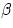Persei) system, where an eclipsing pair (Algol A-B) is accompanied by the third component (Algol C).

### 1. Model Description

The photospheres of eclipsing components are represented by a grid of surface elements, which are treated as plane-parallel and homogeneous ones. In order to calculate the synthetic fluxes of radiation an integration over all surface elements visible at a given orbital phase,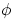, should be done. For this purpose geometrical parameters (i.e., area of elements and their positions) as well as physical ones (temperatures and surface gravities) for all elements have to be known. To describe the system's geometry we assumed the Roche model presented by Kopal (1959) and Limber (1963) for synchronous and nonsynchronous rotation, respectively.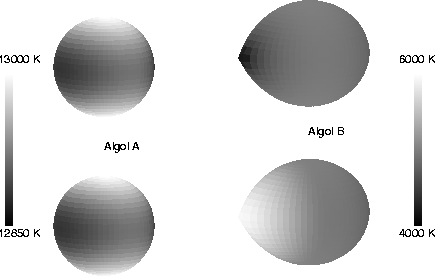Assuming synchronous rotation of the components, the total potential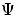can be written as: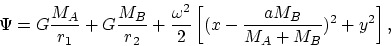(1)
where MA and MB are stellar masses, a - orbital separation of components,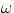- angular velocity of the system about the z-axis, G - the gravitational constant, r12 = x2 + y2 + z2, and r22 = (a-x)2 + y2 + z2. Using spherical polar coordinates (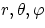)equation (1) can be rewritten as: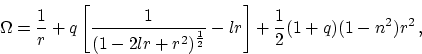(2)
where q=MB/MA,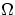is the dimensionless Roche potential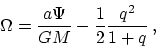(3)
and l, m, n are direction cosines of the radius vector, i.e.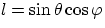,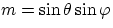,and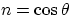.If we assume that the photospheres of primary and secondary components are equipotential surfaces with potentials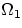and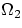, respectively, then the equation (2) allows us to determine the total surfaces of both components. We solve this equation by the iterative Newton-Raphson method.

The local surface gravity follows from the equation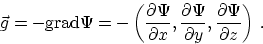If we neglect the effect of irradiation by the companion, the temperature at (i,j)-point of the surface grid depends only on the local value of gravity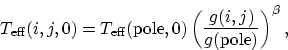(4)
whereis the gravity-darkening exponent. Index 0 refers to effective temperatures in case of neglecting of the irradiation effect (hereafter referred to as intrinsic ones). For photospheres in radiative equilibrium the integral flux is proportional to the local value of gravity (von Zeipel 1924) which implies= 0.25. The gravity-darkening exponent is smaller for photospheres with convection and in case of thick convective envelope= 0.08 (Lucy 1967).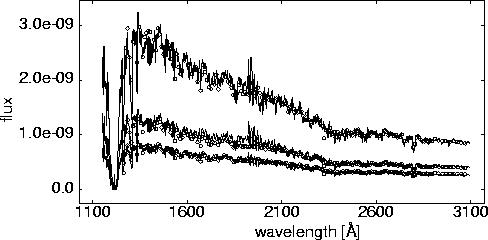If we take into account the irradiation effect, the local temperatures have to be modified. For this purpose an iterative procedure based on the Chen & Rhein (1969) approach was used. In the first step, the intrinsic effective temperatures of both components were set. Then, for each surface element (i,j) of the given component the flux F(i,j) received from the other component was evaluated. The corrected temperature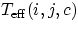follows from the equation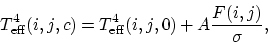(5)
where A is bolometric albedo,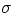- Stefan-Boltzmann constant. A was assumed to be constant over the stellar surfaces. In the case of radiative equilibrium all absorbed energy must be re-emitted; this means that A equals 1.0. For the convective models the bolometric albedo can be smaller than 1.0 and we assume A = 0.5 (cf. Rucinski 1969). The influence of the irradiation effect for the Algol eclipsing pair is shown in Figure 1. Next, we calculate the radiation flux received from the system: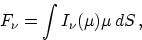(6)
where integration extends over visible parts of all components,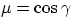(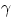is the angle between the surface normal and the line of sight). In this paper we use the second-order limb-darkening law for the evaluation of the specific intensity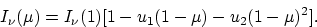(7)
The coefficients u1 and u2, defined by Wade & Rucinski (1985), were calculated for Kurucz's (1996) unpublished models of stellar atmospheres.

### 2. An Example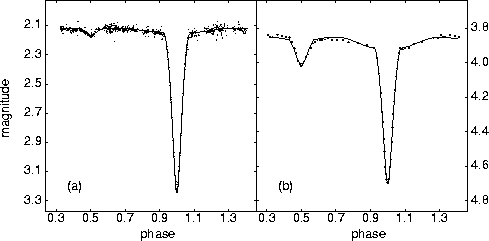We analyse UV spectra of Algol collected by the IUE satellite. We use 15 pairs of IUE spectra from short wavelength primary (SWP) and long wavelength primary (LWP) cameras. We found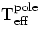(Algol A) = 13000 K,(Algol B) = 5000 K,(Algol C) = 7000 K,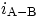=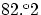and other parameters similar to those given by Tomkin & Lambert (1978). The best-fit solution for the three pairs of spectra is displayed in Figure 2. This model was further verified by UBV observations of Algol taken from Wilson et al. (1972) and by the infrared observations of Chen & Reuning (1966). The comparison of these observations with the theoretical light curves are shown in Figure 3.

### Acknowledgments:

This work was supported by the research grant No. 2 P03D 001 08 from the Polish Scientific Research Committee (KBN).

### References:

Chen, K.-Y., & Reuning, E. G., 1966, AJ, 71, 283

Chen, K. -Y., & Rhein, W. J., 1969, PASP, 81, 387

Kopal, Z., 1959, Close Binary Systems, International Astrophysics Series Vol. 5

Kurucz, R.L., 1996, CD-ROM No.19

Limber, D.N., 1963, ApJ, 138, 1112

Lucy, L.B., 1967, Z. Astrophys., 65, 89

Rucinski, S.M., 1969, Acta Astron., 19, 245

Tomkin, J., & Lambert, D. L., 1978, ApJ, 222, L119

Wade, R. A., & Rucinski, S. M., 1985, A&AS, 60, 471

Wilson, R. E., DeLuccia, M. R., Johnson, K., & Mango, S. A., 1972, ApJ, 177, 191

von Zeipel, H., 1924, MNRAS, 84, 702

© Copyright 1998 Astronomical Society of the Pacific, 390 Ashton Avenue, San Francisco, California 94112, USA

Next: Applications
Up: Computational Astrophysics
Previous: Identification and Analysis of Binary Star Systems using Probability Theory